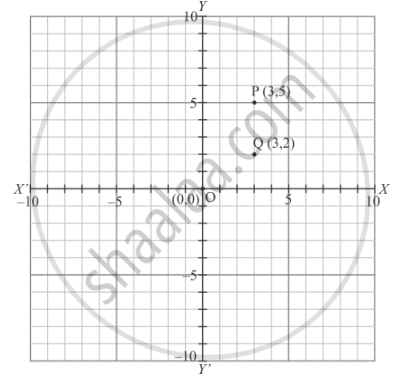# Two Points Having Same Abscissae but Different Ordinate Lie on - Mathematics

MCQ

Two points having same abscissae but different ordinate lie on

#### Options

• x-axis

• y-axis

• a line parallel to y-axis

• a line parallel to x-axis

#### Solution

Let the points  p (3,5)and  Q (3,2) having the same abscissa but different ordinates be shown in the graph given below:Fig: (location of two considered points)

And these points lie on a line parallel to y−axis

Concept: Coordinate Geometry
Is there an error in this question or solution?

#### APPEARS IN

RD Sharma Mathematics for Class 9
Chapter 8 Co-ordinate Geometry
Exercise 8.2 | Q 10 | Page 7

Share